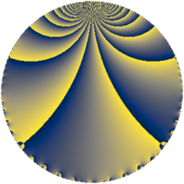# Properties

 Label 76.2.eLevel $76$ Weight $2$ Character orbit 76.e Rep. character $\chi_{76}(45,\cdot)$ Character field $\Q(\zeta_{3})$ Dimension $2$ Newform subspaces $1$ Sturm bound $20$ Trace bound $0$

# Related objects

## Defining parameters

 Level: $$N$$ $$=$$ $$76 = 2^{2} \cdot 19$$ Weight: $$k$$ $$=$$ $$2$$ Character orbit: $$[\chi]$$ $$=$$ 76.e (of order $$3$$ and degree $$2$$) Character conductor: $$\operatorname{cond}(\chi)$$ $$=$$ $$19$$ Character field: $$\Q(\zeta_{3})$$ Newform subspaces: $$1$$ Sturm bound: $$20$$ Trace bound: $$0$$

## Dimensions

The following table gives the dimensions of various subspaces of $$M_{2}(76, [\chi])$$.

Total New Old
Modular forms 26 2 24
Cusp forms 14 2 12
Eisenstein series 12 0 12

## Trace form

 $$2 q + q^{3} + q^{5} + 2 q^{9} + O(q^{10})$$ $$2 q + q^{3} + q^{5} + 2 q^{9} - 8 q^{11} + q^{13} - q^{15} - 3 q^{17} - 8 q^{19} - 5 q^{23} + 4 q^{25} + 10 q^{27} - 7 q^{29} + 8 q^{31} - 4 q^{33} + 20 q^{37} + 2 q^{39} + 5 q^{41} + 5 q^{43} + 4 q^{45} + 7 q^{47} - 14 q^{49} + 3 q^{51} - 11 q^{53} - 4 q^{55} - q^{57} - 3 q^{59} - 11 q^{61} + 2 q^{65} + 3 q^{67} - 10 q^{69} - 11 q^{71} - 15 q^{73} + 8 q^{75} + 13 q^{79} - q^{81} + 3 q^{85} - 14 q^{87} - 3 q^{89} + 4 q^{93} - q^{95} + 5 q^{97} - 8 q^{99} + O(q^{100})$$

## Decomposition of $$S_{2}^{\mathrm{new}}(76, [\chi])$$ into newform subspaces

Label Dim $A$ Field CM Traces $q$-expansion
$a_{2}$ $a_{3}$ $a_{5}$ $a_{7}$
76.2.e.a $2$ $0.607$ $$\Q(\sqrt{-3})$$ None $$0$$ $$1$$ $$1$$ $$0$$ $$q+(1-\zeta_{6})q^{3}+(1-\zeta_{6})q^{5}+2\zeta_{6}q^{9}+\cdots$$

## Decomposition of $$S_{2}^{\mathrm{old}}(76, [\chi])$$ into lower level spaces

$$S_{2}^{\mathrm{old}}(76, [\chi]) \cong$$ $$S_{2}^{\mathrm{new}}(38, [\chi])$$$$^{\oplus 2}$$